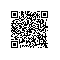# NeHe OpenGL第四十课：绳子的模拟

NeHe OpenGL第四十课：绳子的模拟1. 运动的数学表示
2. 执行模拟的计算机的速度

1. 运动的数学表示:

2. 执行模拟的计算机的速度:

100个点来模拟绳子一段3或4m长的绳子，换句话说，我们的模拟精度就是3到8厘米。

O----O----O----O
1    2    3    4

k: 弹性系数
x: 相距平衡位置的位移

class Spring
{
public:
Mass* mass1;        // 质点1
Mass* mass2;        // 质点2

float springConstant;       // 弹性系数
float springLength;       //弹簧长度
float frictionConstant;       //摩擦系数

Spring(Mass* mass1, Mass* mass2,
// 构造函数
float springConstant, float springLength, float frictionConstant)
{
this->springConstant = springConstant;
this->springLength = springLength;
this->frictionConstant = frictionConstant;

this->mass1 = mass1;
this->mass2 = mass2;
}

void solve()        // 计算各个物体的受力
{
Vector3D springVector = mass1->pos - mass2->pos;

float r = springVector.length();     // 计算两个物体之间的距离

Vector3D force;

if (r != 0)       // 计算力
force += -(springVector / r) * (r - springLength) * springConstant;
...

force += -(mass1->vel - mass2->vel) * frictionConstant;  // 加上摩擦力
mass1->applyForce(force);      // 给物体1施加力
mass2->applyForce(-force);      // 给物体2施加力
}

1. 重力加速度: 9.81 m/s/s 垂直向下
2. 质点个数: 80
3. 相连质点的距离: 5 cm (0.05 meters)
4. 质量: 50 克(0.05 kg)
5. 绳子开始处于垂直状态

f = (绳子质量) * (重力加速度) = (4 kg) * (9.81) ~= 40 N

40 N + (-k * 0.01 meters) = 0

k = 4000 N / m

springFrictionConstant = 0.2 N/(m/s)

1. virtual void init() --->  重置力

2. virtual void solve() --->  计算各个质点的力

3. virtual void simulate(float dt) --->  模拟一次

4. virtual void operate(float dt) --->  执行一次操作

class RopeSimulation : public Simulation     //绳索类
{
public:
Spring** springs;       // 弹簧类结构的数组的指针

Vector3D gravitation;      // 万有引力

Vector3D ropeConnectionPos;      // 绳索的连接点

Vector3D ropeConnectionVel;      //连接点的速度，我们使用这个移动绳子

float groundRepulsionConstant;     //地面的反作用力

float groundFrictionConstant;     //地面的摩擦系数

float groundAbsorptionConstant;     //地面的缓冲力

float groundHeight;      //地面高度

float airFrictionConstant;      //空气的摩擦系数

RopeSimulation(
int numOfMasses,
float m,
float springConstant,
float springLength,
float springFrictionConstant,
Vector3D gravitation,
float airFrictionConstant,
float groundRepulsionConstant,
float groundFrictionConstant,
float groundAbsorptionConstant,
float groundHeight
) : Simulation(numOfMasses, m)
{
this->gravitation = gravitation;

this->airFrictionConstant = airFrictionConstant;

this->groundFrictionConstant = groundFrictionConstant;
this->groundRepulsionConstant = groundRepulsionConstant;
this->groundAbsorptionConstant = groundAbsorptionConstant;
this->groundHeight = groundHeight;

for (int a = 0; a < numOfMasses; ++a)    // 设置质点位置
{
masses[a]->pos.x = a * springLength;
masses[a]->pos.y = 0;
masses[a]->pos.z = 0;
}

springs = new Spring*[numOfMasses - 1];

for (a = 0; a < numOfMasses - 1; ++a)    //创建各个质点之间的模拟弹簧
{
springs[a] = new Spring(masses[a], masses[a + 1],
springConstant, springLength, springFrictionConstant);
}
}

void solve()        // 计算施加给各个质点的力
{
for (int a = 0; a < numOfMasses - 1; ++a)   // 弹簧施加给各个物体的力
{
springs[a]->solve();
}

for (a = 0; a < numOfMasses; ++a)    // 计算各个物体受到的其它的力
{
masses[a]->applyForce(gravitation * masses[a]->m); // 万有引力
// 空气的摩擦力
masses[a]->applyForce(-masses[a]->vel * airFrictionConstant);

if (masses[a]->pos.y < groundHeight)   // 计算地面对质点的作用
{
Vector3D v;

v = masses[a]->vel;    // 返回速度
v.y = 0;     // y方向的速度为0

// 计算地面给质点的力
masses[a]->applyForce(-v * groundFrictionConstant);

v = masses[a]->vel;
v.x = 0;
v.z = 0;

if (v.y < 0)     // 计算地面的缓冲力

masses[a]->applyForce(-v * groundAbsorptionConstant);

// 计算地面的反作用力
Vector3D force = Vector3D(0, groundRepulsionConstant, 0) *
(groundHeight - masses[a]->pos.y);

masses[a]->applyForce(force);   // 施加地面对质点的力
}
}
}

void simulate(float dt)      // 模拟一次
{
Simulation::simulate(dt);     // 调用基类的模拟函数

ropeConnectionPos += ropeConnectionVel * dt;   // 计算绳子的连接点

if (ropeConnectionPos.y < groundHeight)
{
ropeConnectionPos.y = groundHeight;
ropeConnectionVel.y = 0;
}

masses->pos = ropeConnectionPos;    // 更新绳子的连接点和速度
masses->vel = ropeConnectionVel;
}

void setRopeConnectionVel(Vector3D ropeConnectionVel)
{
this->ropeConnectionVel = ropeConnectionVel;
}

RopeSimulation* ropeSimulation =
new RopeSimulation(
80,        // 80 质点
0.05f,        // 每个质点50g
10000.0f,        // 弹性系数
0.05f,        // 质点之间的距离
0.2f,        // 弹簧的内摩擦力
Vector3D(0, -9.81f, 0),      // 万有引力
0.02f,        // 空气摩擦力
100.0f,        // 地面反作用系数
0.2f,        // 地面摩擦系数
2.0f,        // 地面缓冲系数
-1.5f);        // 地面高度

float dt = milliseconds / 1000.0f;      // 经过的秒数

float maxPossible_dt = 0.002f;      // 模拟间隔

int numOfIterations = (int)(dt / maxPossible_dt) + 1;    // 模拟次数
if (numOfIterations != 0)
dt = dt / numOfIterations;

for (int a = 0; a < numOfIterations; ++a)     // 执行模拟
ropeSimulation->operate(dt);

http://nehe.gamedev.net/data/lessons/lesson.asp?lesson=40使用钉钉扫一扫加入圈子
+ 订阅